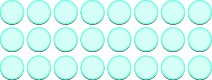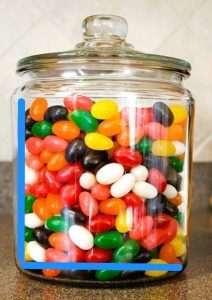## Putting It Together: Whole Numbers

At the beginning of this module, we found Jacquelyn at her birthday party, where she was watching her friends try to guess how many jelly beans were in a jar. There is no “correct” way to estimate the number of jelly beans in the jar, rather, many different methods that will result in similarly close estimates. We can use some of the concepts from this module to help us make an estimate.

One way to find an estimate for the number of jelly beans is to use multiplication. In the module, we modeled multiplication symbolically, as in this example:

To model the product $3\times 8$, we’ll start with a row of $8$ counters.The other factor is $3$, so we’ll make $3$ rows of $8$ counters.Now we can count the result. There are $24$ counters in all.

$3\times 8=24$

We can do a similar thing with the jar of jelly beans, by counting the number of jelly beans up the side of the jar and also along the bottom of the jar.After counting, let’s say that there are 10 jelly beans stacked up the side of the jar and 7 along the bottom of the jar.

Multiply: $7\cdot 10=70$

This tells us that there are about 70 jelly beans facing the front of the jar that we can see.  What about the jelly beans behind them? If we multiply that number facing the front by the depth of the jar, we should get an approximation of the total. We will assume the jar has the same depth as its width, 7 jelly beans. In total, there are 7 layers of the 70 jelly beans facing the front of the jar.

Multiply: $70\cdot 7 = 490$

Does it seem reasonable that the jar will have $490$ jelly beans in it? How would you estimate the number of jelly beans in the jar?

## Contribute!

Did you have an idea for improving this content? We’d love your input.